• Call Now

1800-102-2727•

# Parallax Method: Measuring Distance and Diameter of Nearby Planets & Stars

Can we make huge scales to measure huge distances? Of course not! Then how do we measure large distances like the distance between the Earth and the Sun? We use our mathematical knowledge about triangles called trigonometry (Trigonometry deals with relationships between the lengths of sides and angles of a triangle) to find such large distances.

With the help of the parallax method (which is a special case of triangulation) the distance of far away objects can be measured or estimated. The parallax method has been used throughout the years by astronomers to map the 3D world by observing the 2D sky from the earth.

## Triangulation Method

It is a process of finding out the location of an object/point in space by forming a triangle to the point from given/known points.

## Measurement of Length by Parallax Method

When you hold a pencil in front of you against some specific point on the background (a wall) and look at the pencil first through your left eye O1 (closing the right eye) and then look at the pencil through your right eye O2 (closing the left eye), you would notice that the position of the pencil seems to change with respect to the point on the wall. This is called parallax.

The distance between the two points of observation is the basis for calculation of other lengths, b (O1O2).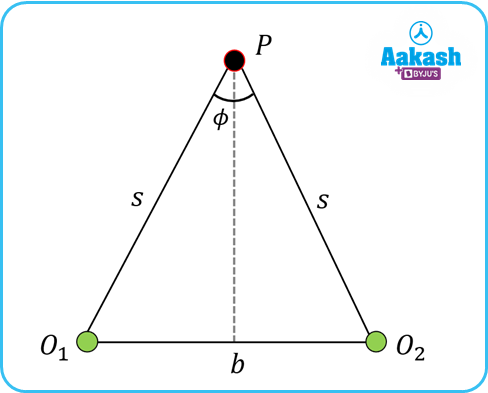Here two points of sight or observers are at a distance, b from each other. They are observing a distant object P. P is very much far from them, so that O1O2 can be considered as an arc of radius s subtending angle ϕ (in radian) at P.

So b=s ϕ

We know the distance between these two points of sight i.e. b and ϕ is measured. From the above equation, we can get the distance, s.

This method is widely used in determining the distance of faraway objects (e.g. stars, planets, ships at the horizon etc).

## Measuring Distance of Nearby Planets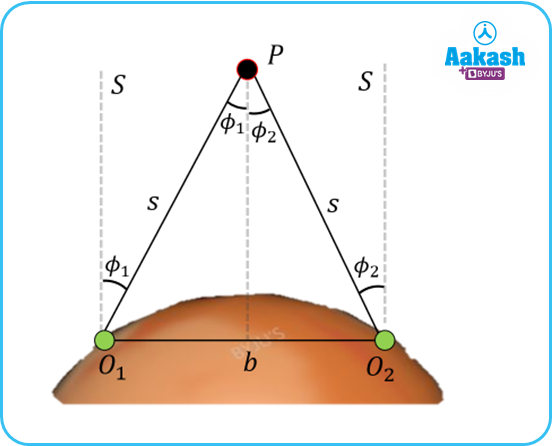Let O1 and O2 are two points of sight on the earth and we are observing a planet P. There is also a star, S far away from the earth which can be treated at same angle of incidence to O1 and O2 i.e. just above head, at 90°.

The planet is observed to be at angles ϕ1 and ϕ2 from O1 and O2 respectively.

The points of sight O1 and O2 are at distance b. The planet is quite far from the earth and can be considered as if it subtends an angle 1+2 with radius s to the points of observations ϕ1and ϕ2

So b=s (ϕ12)

We know the distance between these two points of sight i.e. b and the parallactic angle, ϕ=ϕ12 (in radian) is measured. From the above equation, we can get the distance, s.

## Measuring Diameter of Nearby Planet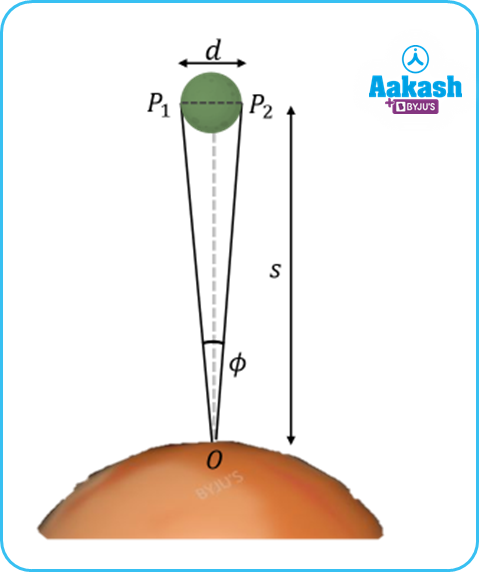From the procedure explained before we can measure the distance of a near planet, s.

Now, from an observatory point, O we measure the angle subtended by the planet i.e. ϕ.

So, P1P2=d where d is the diameter of the planet.

So, d=sϕ

Thus, we can measure the diameter of a nearby planet using the parallax method.

## Measuring Distance of Nearby StarFor measuring the distance of nearby star S1, we have to take a faraway star S2 as reference whose angle of incidence on earth can be treated as constant over the year i.e. above head or 90°.

We are to measure the distance, d of the nearby star from the sun. For that, we measure the angle made by S1 with respect to S2 at two extremes of earth orbit. Let those be ϕ1and ϕ2 respectively. We know the diameter of the earth’s orbit, D.

As S1is quite far from the sun, the diameter of the earth’s orbit can be taken as an arc with an angle ϕ12 subtended at S1. So, the distance d can be measured as,

D=d(ϕ12)

1 LY= 9.461×1015 m

## Parsec (pc)Parsec is a unit of length which is used to measure large distances mainly for astronomical objects outside the solar system. It is approximately equal to 3.26 LY or 206×103AU. It is defined as the distance at which one astronomical unit subtends an angle of one arc second. The nearest star Proxima Centauri is about 1.3 pc.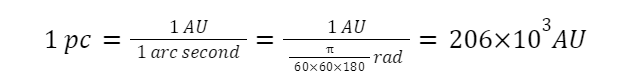## Practice Problems of Parallax Method

Question.1 From a space observatory satellite near earth, the planet Saturn subtends an visual angle of 77 10-3 radian. From doppler test it is found that the distance from the satellite to Saturn is 1.5107 109 km. What is the diameter of Saturn?

Answer. Here, d=sϕ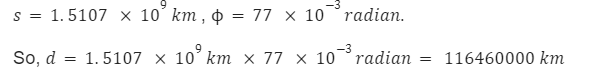Question.2 The sun’s angular diameter is measured to be 1920''. The distance of the sun from the earth is 1.496×1011 m. What is the diameter of the sun?

Answer.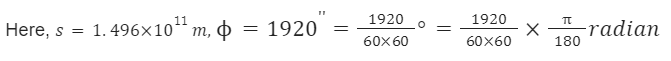So, d=sϕ=13.92×108 m

Question.3 The moon is observed from points A and B on the earth, A and B are diametrically opposite to each other. The angle subtended at the moon by the two directions of observation is 1°54' .Given the diameter of the earth to be about 1.276×107 m ,compute the distance of the moon from the earth.

Answer. Here,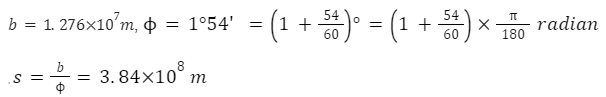Question.4 A man observes a speed boat at the horizon (5 km away). The speed boat covers an angular displacement of 0.04 radian in 10 s. What is the speed of that speed-boat ?

Answer.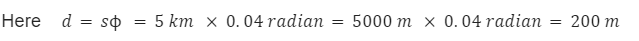The speed boat covers 200 m in 10 s.

So the speed will be 200 m / 10 s = 20 m/s

## FAQs of Parallax Method

Question.1 Measuring distance of nearby star by parallax method is accurate when the distance of the star is

1. greater than 10 LY
2. less than 10 LY
3. greater than 100 LY
4. less than 100 LY

Question.2 Parsec is the distance which subtends an angle of

1. 1 degree
3. 1 second
4. none of the above

Question.3 Earth’s orbit around the sun has radius nearly,

1. 1 LY
2. 1 AU
3. 1 pc
4. none of the above

Question.4 Very large distance can be measured by,

1. Least count method
2. Parallax method
3. inch tape
4. none of the above